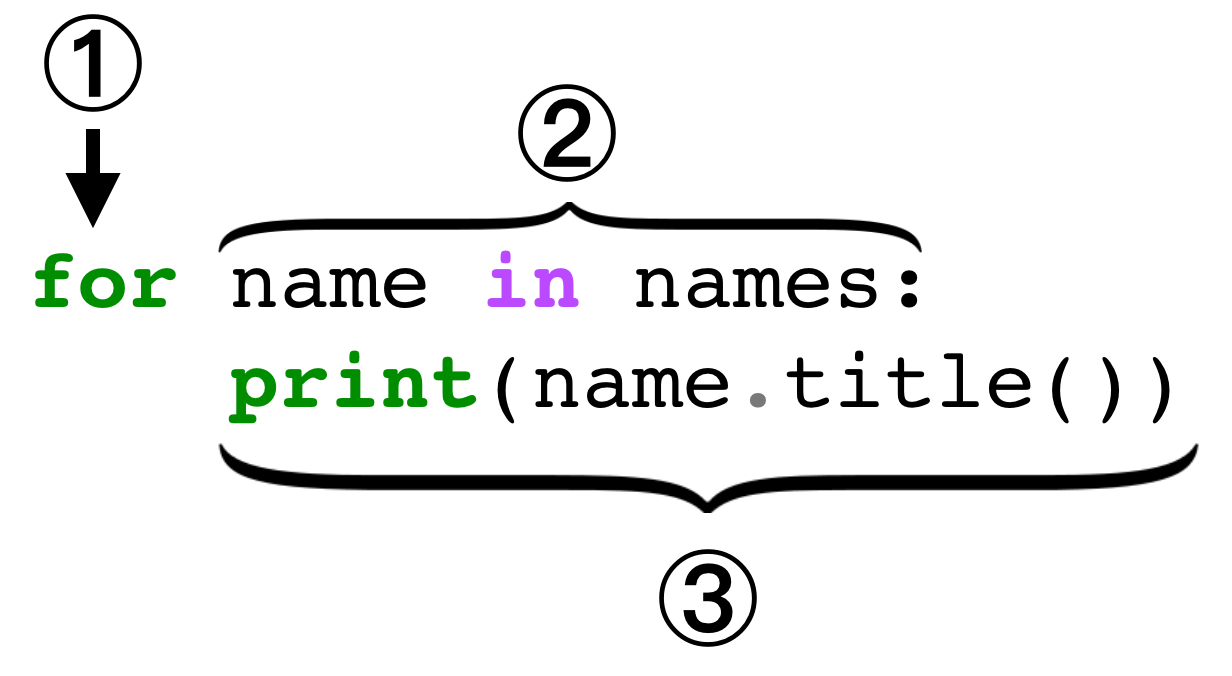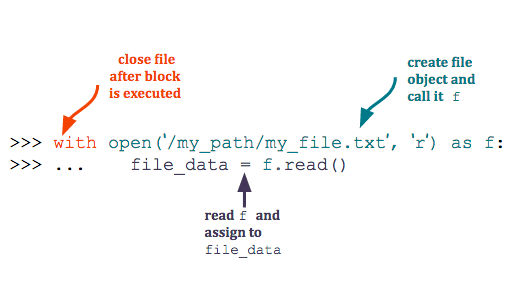# Python 3 Syntax – Udacity

``````def readable_timedelta(days):
"""Print the number of weeks and days in a number of days."""
#to get the number of weeks we use integer division
weeks = days // 7
#to get the number of days that remain we use %, the modulus operator
remainder = days % 7
return "{} week(s) and {} day(s)".format(weeks, remainder)

``````````````````